formatio reticularisSCIENTIFIC SOFTWARE AND SERVICES FOR RESEARCH IN SYSTEMS BIOLOGY AND MEDICAL CYBERNETICS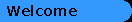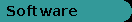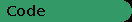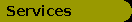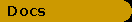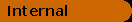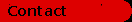CodeA function plotter in R:

With the statistical language R, it is very easy to write a simple function plotter in a few lines. Simply enter the following code in a script window:

``````# simple function plotter in R #

x <- seq(from=0, to=10, by=0.1);
y <- seq(from=0, to=5, by=0.05);
plot(x,y,type="n");
G <- 5;
D <- 2;
lines(x, G*x/(D+x));``````

``````

Then modify the settings, e. g. coordinates and of course the function (the second argument of the "lines" function in the last line) as desired and execute the script. In the example, a Michaelis-Menten function with maximal output G (e. g. velocity or receptor density) and dissociation constant D is drawn.

The result will be as follows: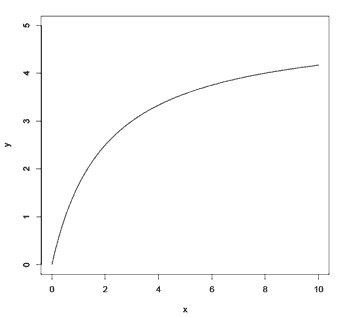© 1999 - 2018 J. W. Dietrich
Imprint and legal information | Privacy policy | Last revision: Fri, Jun 1, 2018